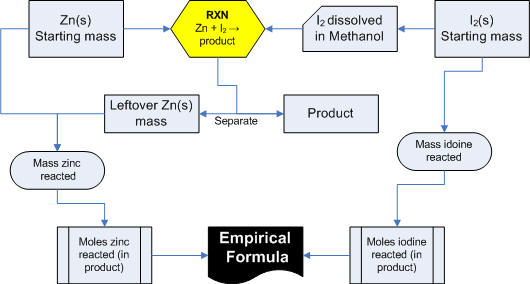Zinc Iodide, what is the formula?

In this experiment, you will combine elemental zinc (Zn) and iodine (I2) to form “zinc iodide.”  You will determine the empirical formula of your “zinc iodide” by determining how much zinc and iodine has reacted.

A partial reaction is shown below:
___ Zn(s) + ___ I2 (s)  ___ Zn?I?

If you don’t know what ratio of zinc to iodine is correct, how can you plan your experiment?  In this case, we will use enough zinc to make certain that zinc will be in excess.  In other words, when all the iodine has reacted, there will still be zinc metal left over.  Iodine is the limiting reactant.  The extra shiny, silver-colored zinc will be removed from the reaction; its mass will not be included in the calculation of the empirical formula.

We need the molecular formula of zinc iodide. The only information we have which would be of help to you is that the empirical formula can be found through the mole ratio of iodine and zinc.

7 min screencast description of experiment

The lab report will consist of a reasonably detailed procedure (don't forget the calculations that you did, they are part of your procedure), clean well-labeled table that includes all raw data as well as all calculated values (including the mole ratio (actual! reported to 3 sig figs!) and the empirical formula you obtain) Below the data table give one sample of each calculation.

You also need to include a short form memo type 'commentary' section.

What we know:
1. Mixing the two solids will never be an effective method for making the reaction occur.
2. Iodine and the zinc iodide product dissolve in methanol, zinc does not.
3. The empirical formula for zinc iodide is the same as the molecular formula.
4. The balanced chemical equation consists of stoichiometric factors which relate the number of moles of each species, not the mass ratios.
5. Figuring out the molar ratios of Zn and I in the product is as simple as knowing how much of each reactant reacted. You can then get a mass ratio, which you can convert into a mole ratio.
6. This is definitely a limiting reagent type problem. You will have to add excess of one reactant, and determine how much of EACH reactant reacted.

A picture of the experiment:Prelab Questions:
1. An experiment is done where 1.054 g of copper reacted with 0.133 g of oxygen to form 1.187 g of copper oxide. Determine the empirical formula of copper oxide. Show your work, of course.
2. Review the MSDS for solid Zn and solid I2 and briefly summarize the hazards.
3.Construct a table for the necessary information needed. (on another piece of paper, show instructor upon entering lab)

'Hints' from student who have done this lab before:
- Think about what information the molecular formula gives; and what type of data you need to calculate it.
- Don't weigh hot things on the analytical balances.
- Don't take your time, or you will not get done.
- Don't add too much methanol.

Last Edited 9 Oct 2007